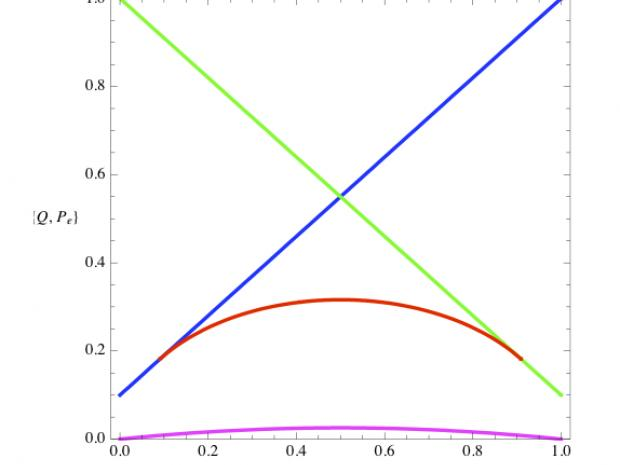Events

# Physics Seminar - Discrimination of quantum states with selected applications

For NYU Community### Janós Bergou, Professor of Physics, CUNY Hunter College

In the emerging field of quantum information processing the carrier of information is a quantum system and the information is the quantum state of the system itself. The main means of processing the information is to perform various transformations on the state in order to produce a desired target state. The problem is in the read-out of this information: as every textbook on quantum mechanics tells us, the state of a quantum system is not an observable. How to access the information by measurements is the subject of State Discrimination, which, thus, constitutes the read-out stage of quantum information processing. Optimized discrimination strategies often lead to generalized measurements (Positive Operator Valued Measures, POVMs). Review of recent progress in state discrimination, implementation of the optimal POVMs by linear optics and selected applications will be discussed.

FIGURE: Even if we know for sure that our system is prepared in one of only two known states, to determine unambiguously which of the two possible states is the actual state of the system is a difficult task. The probability Q of failing to identify the state is plotted vs. probability η1 that the first of the two states was prepared. Green line: Q for a standard quantum measurement that sometimes identifies the first state. Blue line: Q for a standard quantum measurement that sometimes identifies the second state. Red line: Q of the optimal measurement (optimal POVM). Purple line: If errors are permitted, the optimal measurement is the one that minimizes the probability P of erroneously identifying the state. Green line is the minimum error probability P.